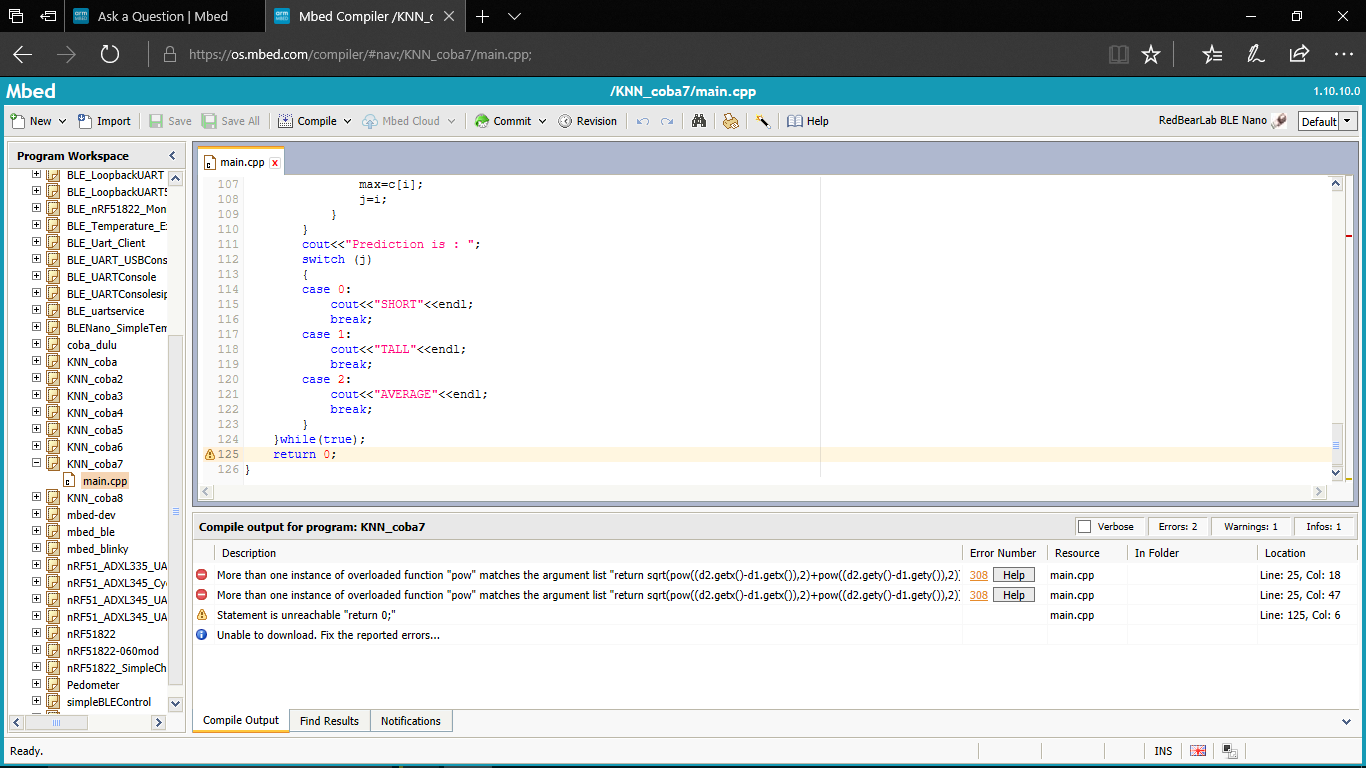## How to solve this KNN for implementing to nRF51822

Hello world..

I wanna share my progress for implementing KNN for nRF51822 and there's the code that I compiled it to mbed compiler..

#### include the mbed library with this snippet

```#include<iostream>
#include<math.h>
#include<stdlib.h>
#define MAX 20
#define k 4
using namespace std;
enum category{SHORT,TALL,AVERAGE};
class data{
int x,y;
category cat;
public:
void setd(int a,int b,category c){
x=a;
y=b;
cat=c;
}
int getx(){return x;}
int gety(){return y;}
category getcat(){
return cat;
}
};//end of class
int dis(data d1,data d2)
{
return sqrt(pow((d2.getx()-d1.getx()),2)+pow((d2.gety()-d1.gety()),2));
}
int main()
{
do{
int p,q; //input
int a[MAX]; //store distances
int b[k]; //to get min distances, used in calc
int c[k]; //to store freq
for(int i=0;i<k;i++){ //initiLIZATION
b[i]=-1;
c[i]=0;
} int min=1000;
cout<<"Enter x,y(negative value to exit): ";
cin>>p>>q;
if((p < 0) | (q < 0))
exit(0);
data n; //data point to classify
n.setd(p,q,SHORT);
data d[MAX]; //training set

d.setd(1,1,SHORT);
d.setd(1,2,SHORT);
d.setd(1,3,SHORT);
d.setd(1,4,SHORT);
d.setd(1,5,SHORT);
d.setd(1,6,SHORT);
d.setd(1,7,SHORT);
d.setd(2,1,SHORT);
d.setd(2,2,SHORT);
d.setd(2,3,AVERAGE);
d.setd(2,4,AVERAGE);
d.setd(2,5,AVERAGE);
d.setd(2,6,AVERAGE);
d.setd(2,7,AVERAGE);
d.setd(5,1,TALL);
d.setd(5,2,TALL);
d.setd(5,3,TALL);
d.setd(5,4,TALL);
d.setd(5,5,TALL);
d.setd(5,6,TALL);
for(int i=0;i<20;i++){
a[i]=dis(n,d[i]);
cout<<"\t\t"<<a[i]<<endl;
}
//k-nearest neighbours calculation i.e smallest k distances
for(int j=0;j<k;j++)
{
min=1000;
for(int i=0;i<20;i++)
{
if(i!=b&&i!=b&&i!=b)
{
if((a[i]<=min))
{
min=a[i];
b[j]=i;
}
}
}
cout<<min<<endl;
}
//counting frequency of a class in each neighbour
for(int i=0;i<k;i++)
{
switch (d[b[i]].getcat())
{
case SHORT:
c++;
break;
case AVERAGE:
c++;
break;
case TALL:
c++;
break;
}
} //counting max frequency
int max=-1,j;
for(int i=0;i<k;i++)
{
if(c[i]>max){
max=c[i];
j=i;
}
}
cout<<"Prediction is : ";
switch (j)
{
case 0:
cout<<"SHORT"<<endl;
break;
case 1:
cout<<"TALL"<<endl;
break;
case 2:
cout<<"AVERAGE"<<endl;
break;
}
}while(true);
return 0;
}
```

but there was several problem that show like this one..

#### Quote:

Error: More than one instance of overloaded function "pow" matches the argument list in "main.cpp", Line: 25, Col: 18

Error: More than one instance of overloaded function "pow" matches the argument list in "main.cpp", Line: 25, Col: 47

Warning: Statement is unreachable in "main.cpp", Line: 125, Col: 6best regards for you to help me.. thanks..

## 1 Answer

##### 3 years, 1 month ago.

The pow function is expecting float/double data types and you are loading with int types pow is an overloaded function and the compiler doesn't know which way to use the integers whether they should be floats/doubles/long doubles hence the error change line 25

```    return sqrt(pow((d2.getx()-d1.getx()),2)+pow((d2.gety()-d1.gety()),2));
```

to

```    return sqrt(pow(((double)d2.getx()-(double)d1.getx()),2.0)+pow(((double)d2.gety()-(double)d1.gety()),2.0));
```

or

```    return sqrt(pow((double)(d2.getx()-d1.getx()),2.0)+pow((double)(d2.gety()-d1.gety()),2.0));
```

then you will be 'casting' the integers into double and the overloaded function will work

NB the integer litteral constant '2' has been changed to '2.0' to indicate this is also a double

Accepted Answer